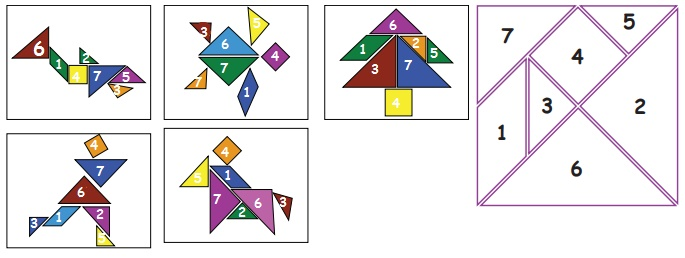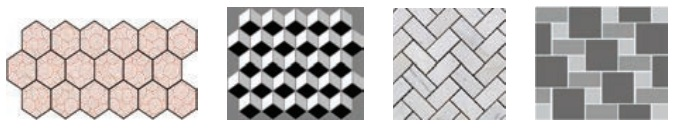Home | | Maths 3rd Std | Tangram

# Tangram

Tangram is a traditional Chinese puzzle made of a square divided into seven pieces (one parallelogram, one square and five triangles) that can be arranged to match particular designs.

Tangram

Create shapes using tangram pieces

Tangram is a traditional Chinese puzzle made of a square divided into seven pieces (one parallelogram, one square and five triangles) that can be arranged to match particular designs. We can make many figures of animals people and other things. Simplified version of tangram puzzle is available with five pieces also.

5 pieces tangram

look at 5 pieces of the tangram. cut the 5 pieces from a paper with help of your elders and try make the given shapes out of it.Try this

How many triangles are there in a five piece tangram set? Are they equal in size?

There are three triangles. 2 and 5 equal in size, but 1 is different in size.

7–pieces tangram

Here is the picture of a seven–piece tangram. You can cut out these pieces from a paper and put them together in different ways to make some very interesting shapes.

Teacher’s note: Teacher can enable the children create various shapes using the tangram sets.Practice

Number the pieces in each of these figures according to the numbering in the tangram puzzle.Tile a given region using a tile of given shape.

When you fit individual tiles together with no gaps or overlaps to fill a flat space, you have a tiled floor.

Observe the picturesBaked clay in the form of a shape that is used to cover surfaces is called tile.

Let us Explore

There are some shapes that cannot be used to make tiling patterns why? what are they? Name them and give reasons.Answer: opposite side or diagonals must be equal for tiles to join. Some shapes that cannot be used to make tiling patterns are Circles or ovals. Both have no opposite side to joint.

Practice

Complete the following tiling pattern.When we repeat individual tiles or patterns together with no gaps or overlaps to fill a flat space, the arrangement is called tessellation (or tiling).

Activity 6

Tessellate a new region using the following shapes:Triangles, squares, hexagons are the regular polygons tessellate in the plane.

HereDistinguish between shapes that tile and that do not tile.

Observe the following pictures that do not tile.Though pentagons and heptagons are regular polygons they do not tessellate.

Activity 7

Ask the children to draw tessellate in the plane region using square and pentagon.Tags : Geometry | Term 1 Chapter 1 | 3rd Maths , 3rd Maths : Term 1 Unit 1 : Geometry
Study Material, Lecturing Notes, Assignment, Reference, Wiki description explanation, brief detail
3rd Maths : Term 1 Unit 1 : Geometry : Tangram | Geometry | Term 1 Chapter 1 | 3rd Maths